# Best Calculators For College Algebra

Algebra is one of major sections of college math, and every student will have to take at one point or the other. A major tool to aid the learning of and calculations in algebra classes is a scientific calculator that can perform basic algebraic calculations. Since having a calculator is expedient for every student, knowing how to choose the right one that will be efficient for learning is important. In this post, we will discuss some of the tips for choosing the best calculators for college algebra and will also itemize some of the best algebra calculators you can purchase.

## 1. Texas Instruments TI-36X Pro Engineering/Scientific CalculatorComing first on the list of best calculators for college algebra, Texas instrument calculators are known to have the best features of all scientific calculators. The TI-36X pro is ideal for curricula in which graphing technology is not permitted. It has a Multiview display that can show several calculations at the same time; is that cool? With its MathPrint, you get math expressions, symbols, and stacked fractions displayed as you will have seen them in your textbook.

## 2. Texas Instruments TI-30XS MultiView Scientific Calculator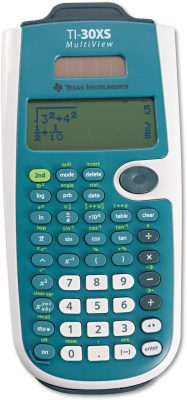This is yet another model by Texas Instrument calculator; known for producing the best calculators for college algebra. The TI-30XS model is can perform multiple calculations, compare results, and explore patterns on the same screen. You also get the MathPrint feature with this model. If while calculating you need to scroll through previous entries and investigate critical patterns, you can do that with the TI-30XS. You can also paste new calculations into an existing entry.

## 3. Sharp EL-W516TBSL 16-Digit Advanced Scientific Calculator with WriteView 4 Line DisplaySharp has remained a leader in the electronic calculator industry. Its EL-W516TBSL model is a large 16 digit, four-line LCD. It has a Writeview display ability that allows you to view math expressions the way you would see them in textbooks. It is approved for SAT, PSAT/NMSQT, AP exams, Math IC, etc. Talking about the hardware, this calculator is built to last for years; a student can use it from the start of high school till college.

## 4. Texas Instruments TI-84 PLUS CE Graphing Calculator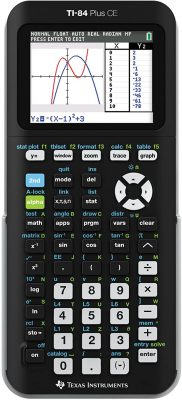Yet another pick when talking about the best algebra calculators, is the TI-84 PLUS CE graphing calculator. This model can import and use images for calculation. If you have a graph image for example, and you do not want to spend too much time solving it, you can easily load such an image on this calculator. It enhances readability with high resolution, backlit display. It also makes graphs easier to read by adding grid lines. It is more than enough for calculations in Algebra I.

## 5. Texas Instruments TI-Nspire CX II CAS Color Graphing Calculator with Student SoftwareIf you are looking for new features in calculators for algebra 1, this model will meet your expectation. Some of its new features are label axes scales which create a visual context that promotes understanding; TI-Basic programming enhancement for a visual illustration of key math, science, and STEM ideas; new app icons, color-coded screen tabs, etc. The TI-Nspire CX II CAS color graphing calculator has up to six graph styles, and fifteen colors to select from for differentiating the look of each graph.

## 6. Casio fx-9750GII Graphing CalculatorMore students in college will be familiar with any of Casio’s calculators for algebra 1. It is one of the affordable calculators for college learning. The Casio fx-9750GH calculator does quick pie charts and bar graphs. It has a USB port through which you can transfer a file to it. You can easily import graphs into the calculator. This graphing calculator has a high-resolution LCD with intuitive software design. It is suitable for students from middle school till college.

## 7. Texas Instruments TI-34 MultiView Scientific Calculator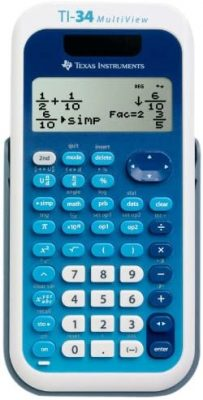Still exploring the best algebra calculators, we have TI-34 Multiview scientific calculator. It has a 4-lines display which enables scrolling and editing previous entries. This model has the same functionality as the TI-34II explorer plus display and feature enhancements. You can easily access all mode settings; you can easily read and navigate pull-down menus. It is suitable for all algebra I calculations; it does not lack any operations you need it to do for solving questions under algebra I.

## 8. Texas Instruments TI30XIISWHITE 2-Line Scientific Calculator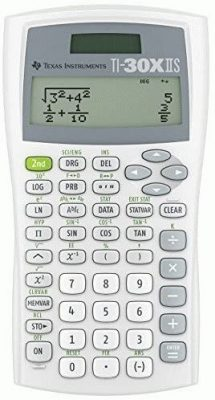This model of Texas instrument calculator has a 2-line display that shows entry and calculated results at the same time. It easily handles 1 and 2 variable statistical calculations, and three angle modes (degrees, radians, grads). Five variables can also be assigned to their memory slots. You can easily navigate through your previous entries, using the four arrow keys. It is ideal for general math, pre-algebra, algebra 1 and 2, geometry, and so on.

## 9. Casio FX115ESPLUS Scientific Calculator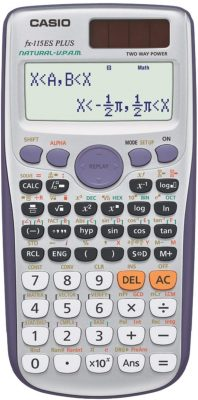Coming back to one of the popular scientific calculators, the Casio fx-115ES PLUS has a natural textbook display that shows results as you will have it in your textbooks. It has over 280 functions which include, statistics, base arithmetic, fractions, complex number calculations, standard deviation, linear regression, polar-rectangular conversions, and so on. It also has a multi-replay function that allows the user to check through calculations. You can edit expressions and recalculate answers.

## 10. Casio Scientific Calculator Black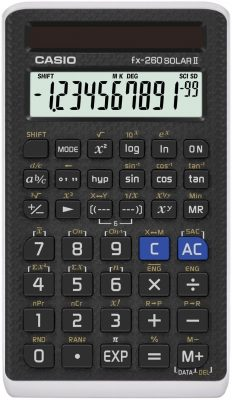This is the very last on our list of best college algebra calculators. This calculator deserves a place on this list mainly because of its simplicity. It performs basic algebra 1 functions such as logarithms/exponents, fractions, and trig functions. College students can easily tackle math formulas with this versatile calculator. This Casio scientific calculator has about 144 functions which include fraction, trigonometry, and fixed decimal capability to take on diverse equations.

## Buying Guide For Best Calculators For College Algebra

Type of calculator: There are different calculators made to handle different levels of math operations. The types of calculator available are:

• Scientific calculator: This is the most suitable of all types for algebra classes. It can be used for calculations when solving different algebraic functions. Such calculators can handle functions such as quadratics, inequalities, exponents, and so on.
• Graphing calculator: This is an advanced kind of calculator. It handles complex functions and topics in math. You can choose to use it for algebraic functions but, it is costly than the usual scientific calculator for algebra.
• Basic calculator: This is ideal for lower-class calculations. It only performs calculations such as addition, subtraction, finding root, and other basic math operations. This type of calculator is not ideal for algebra.

Features: Various calculators can be used for algebra but, the features each possesses sets the difference. When choosing your calculator, you should choose calculators with better features such as solar and battery-powered, sleek design, Multiview display, USB port to import images, and so on. There are some scientific calculators that ideal for algebra but can also be used for graphing due to an extra feature.

Topics: Let’s discuss some algebra topics and see why you will need a calculator.

• Linear equations: This topic involves lots of fractions, decimals, integer coefficients, and a lot more. A student learns the relationship between linear equations, proportional relationships, and lines.
• Expression: Here, a student will need to translate word problems into algebraic expression and interpret the expression structure. Much time will be taken trying to translate and understand the expression, therefore, requiring the student to finish up with the use of a calculator.
• Polynomial: In this segment, students learn how to solve higher-order equations. Students learn to factor in rules relating to addition, subtraction, and multiplication into polynomials. They also learn the zero-factor property.
• Quadratic equations: This topic involves lots of square root property, completing the squares, and quadratic formulas. A calculator will truly be of help in such a class.
• Functions: This topic teaches students to recognize, interpret, and evaluate different types of functions. They also learn how to determine intercepts, domain, and range.

## Conclusion

The calculators listed above can be used to perform various math operations. Before you decide on the calculator to pick, know the task you need to perform, and know the ability of the product you are about to purchase. For best performance and excellence, Texas Instruments TI-Nspire CX II CAS Color Graphing Calculator ranks best of the best calculators for college algebra.

We will be happy to hear your thoughts